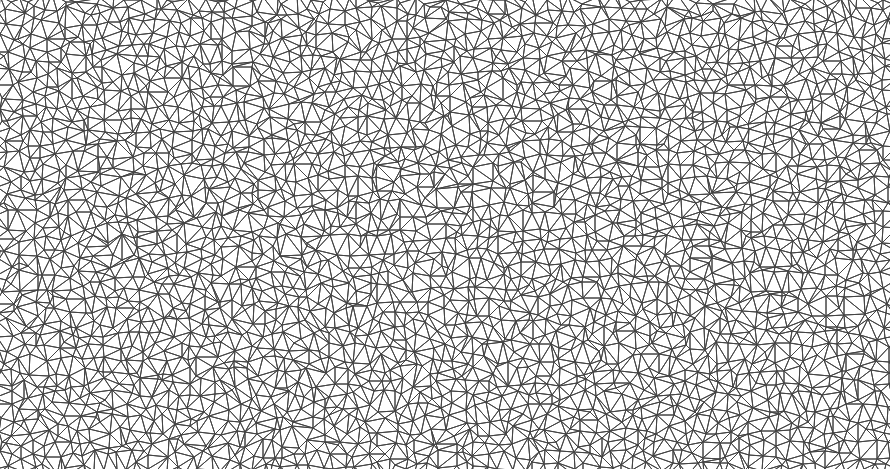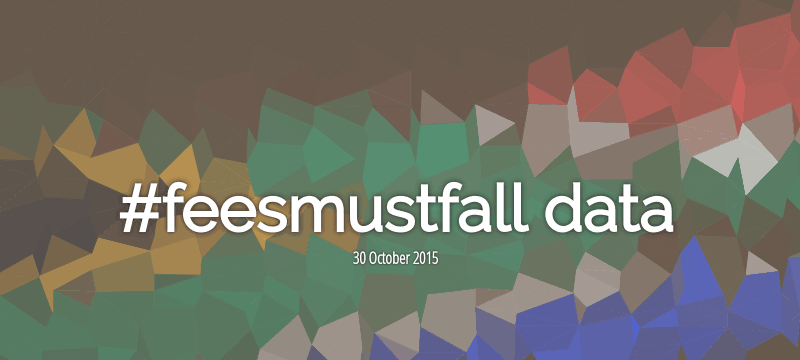# Delaunay Triangles

## Increasing Perceived Speed with Delaunay Triangles

I've been on a bit of a performance hunt lately on this site - key points being the move over to PHP7, nginx, and http2 (post on this coming soonish). All of those changes came with fairly good gains on the server side.

For the client side, besides the usual "make sure you cache everything", I'm using a JavaScript library (http://instantclick.io/)  to preload content on mouseover. Yes, this hits the server a bit if users wiggle around too much, but it's negligible for a blog and offers a nice feeling of speed between clicks.

One of the last points that has been nagging at me is how to reduce the time it takes to display a bit of colour on the page. With most of the colour coming from images, there isn't much option here besides heavy compression (lossy) or getting faster internet. And because we don't have complete control over internet speeds - compressing the image is what we're going to do.

Now for this post's main point: Increasing Perceived Speed with Delaunay Triangles. What does that mean? "Perceived Speed" is how fast you think the site is, and we're going to increase it. Delauney Triangulation (example below) is a geeky bit of maths that I won't cover here - go read the wikipedia article if you're interested in the nuts and bolts. We're using it as a way to "compress" an image down to something that still looks familiar (and a bit fancier than a gradient/single colour).Simple Delaunay triangles based off of a set of random points

## How it works

We'll set up a bit of code (bottom of the page) that figures out all the triangles and colours and what not. Once that's working we can produce images like the following:

And then when an image is displayed, we almost instantly draw the "compressed" image (here's that perceived speed) while we wait for the source image to finish downloading. Once it's complete, we switch it in - and because it's now cached, this process shouldn't happen too often.

## Final Result

If you didn't notice it on this page, it's either because your internet is fast (I oversized the banner image in an attempt to make it noticable) - or you weren't paying attention! If you'd like to test it out, clear your cache and refresh this page. Or just look at the following example:An example of an image when throttling the network connection to mobile (3G) speeds (14KB vs 649KB)

## Downsides

Because we're sending the "compressed" image and the source image down to the user, we are going to use more data overall. The tradeoff is worth it (opinion), and the size of the compressed images is controllable to a point. For example, lower it down to ~20 blocks and you'll still see something resembling the source image, all in a few KB.

There's also the fact that it requires JavaScript.

And of course, there's the fact that a lot of this is a bit silly - the preload images could be generated on the server side (vastly outperforming our method of sending down the point data as text). But this was fun.

## The code

### Server side:

Passing an image path to this function will return an array of points ([x, y, colour]) that the client side code will expect. I've simplified it quite a bit to get the core idea across (i.e. the site has extra functionality). Blocksize is how large each "pixel" is (higher = less points, lower filesize). Variance is how accurate each point's position should be - adding a bit of randomness to each image.

```function getPointsAndColours(\$image_path, \$gridsize = 50, \$variance = 5){

//lazy way of opening the source image
\$image = imagecreatefromstring(file_get_contents(\$image_path));

\$width  = imagesx(\$image);
\$height = imagesy(\$image);

//snap the width/height by rounding up to the nearest gridsize multiple
\$width  = \$width  + \$gridsize - (\$width  % \$gridsize);
\$height = \$height + \$gridsize - (\$height % \$gridsize);

\$points = [];

//Loop over the image and populate the points
//We add a bit of variance to make each copy unique
//(0 variance will pixelate/create squares)
for(\$x = 0; \$x <= \$width; \$x+=\$gridsize){
for(\$y = 0; \$y <= \$height; \$y+=\$gridsize){
\$pixel = imagecreatetruecolor(1,1);

//A cheap way get the average colour of the block
//We just resample the block to 1x1 and let the resampler worry about it,
//After resizing the pixel colour will always be the average colour.
imagecopyresampled(\$pixel, \$image, 0, 0, \$x, \$y, 1, 1, \$gridsize, \$gridsize);
\$rgb = imagecolorat(\$pixel, 0, 0);
\$r   = (\$rgb >> 16) & 0xFF;
\$g   = (\$rgb >> 8) & 0xFF;
\$b   = \$rgb & 0xFF;
imagedestroy(\$pixel);

\$colour   = sprintf("#%02x%02x%02x",\$r,\$g,\$b);
\$points[] = array(\$x + (rand(-\$variance,\$variance)),\$y + rand(-\$variance,\$variance), \$colour);
}
}

return \$points;
}```

### Client Side:

On the client side, we take the point array generated on the server and use it to generate the preload image. The script will work on all elements that have the class "triangles". Any sort of validation / error checking left as an exercise ;)

Below is an example of the required HTML (this page's banner), followed by the JavaScript

Makes use of the Fast Delaunay library from here: https://github.com/ironwallaby/delaunay

```<div class="post_header triangles"
data-width="936"
data-height="468"
data-points=" <--snip--> ">
</div>```
```var delaunay = Delaunay;

//Find all .triangles
var triangleImages = document.getElementsByClassName('triangles');

//Where we'll hold references to the source images
var fullImages = [];

for(var idx = 0; idx < triangleImages.length; idx++){
var triangleImage = triangleImages[idx];
var imageSrc = triangleImage.dataset.src;
var points = triangleImage.dataset.points;
points = eval(points); //filthy, I know.

var pointsWidth = triangleImage.dataset.width;
var pointsHeight = triangleImage.dataset.height;

//Scale the points up to meet the container's size
//This needs a bit of work (crop/aspect ratio etc)
//But the nifty thing is the triangles scale like a
//vector image.
scale = (triangleImage.offsetWidth) / pointsWidth;
points.forEach(function(point,idx){
points[idx] = points[idx] * scale;
});

//Scale the height if we have it, as above.
if(pointsHeight !== undefined){
scale = (triangleImage.offsetHeight+50) / pointsHeight;
points.forEach(function(point,idx){
points[idx] = points[idx] * scale;
});
}

//Setup a canvas and resize it to fit it's container
var canvas = document.createElement('canvas');
var ctx = canvas.getContext('2d');
canvas.width = triangleImage.offsetWidth;
canvas.height = triangleImage.offsetHeight;

//The magic happens here
var triangle_indices = delaunay.triangulate(points);
var triangles = [];

var lookup_point = function(i){ return points[i]; };
for (var i=0; i < triangle_indices.length; i += 3) {
var vertices = [triangle_indices[i], triangle_indices[i+1], triangle_indices[i+2]].map(lookup_point);
triangles.push(vertices);
}

//Draw all the triangles.
triangles.forEach(function(triangle){
ctx.fillStyle = ctx.strokeStyle = triangle;
ctx.beginPath();
ctx.moveTo.apply(ctx, triangle);
ctx.lineTo.apply(ctx, triangle);
ctx.lineTo.apply(ctx, triangle);
ctx.closePath();
ctx.fill();
ctx.stroke();
});

//Grab the final image and set it to the background
//image of the container.
data = canvas.toDataURL();
triangleImage.style.backgroundImage = 'url(' + data + ')';